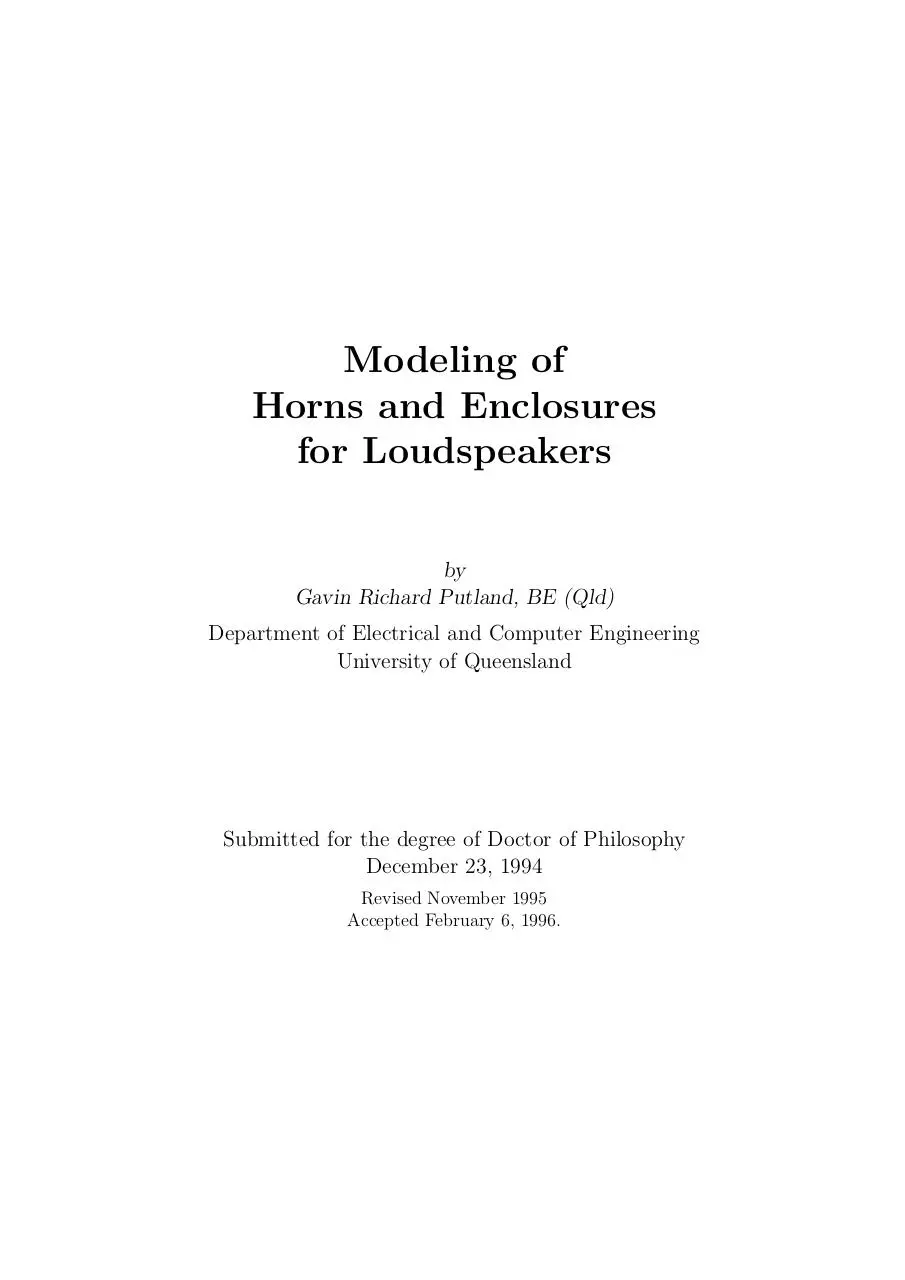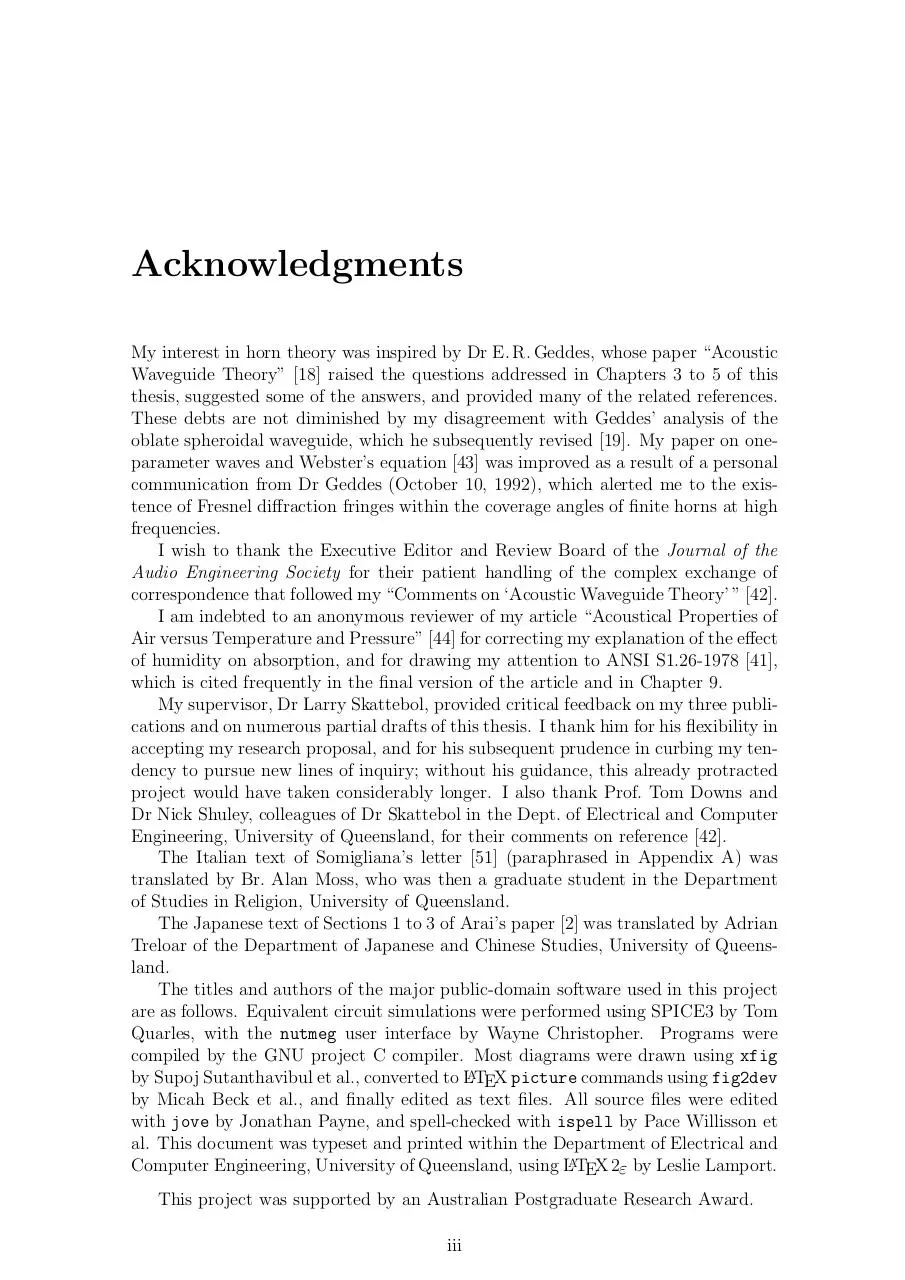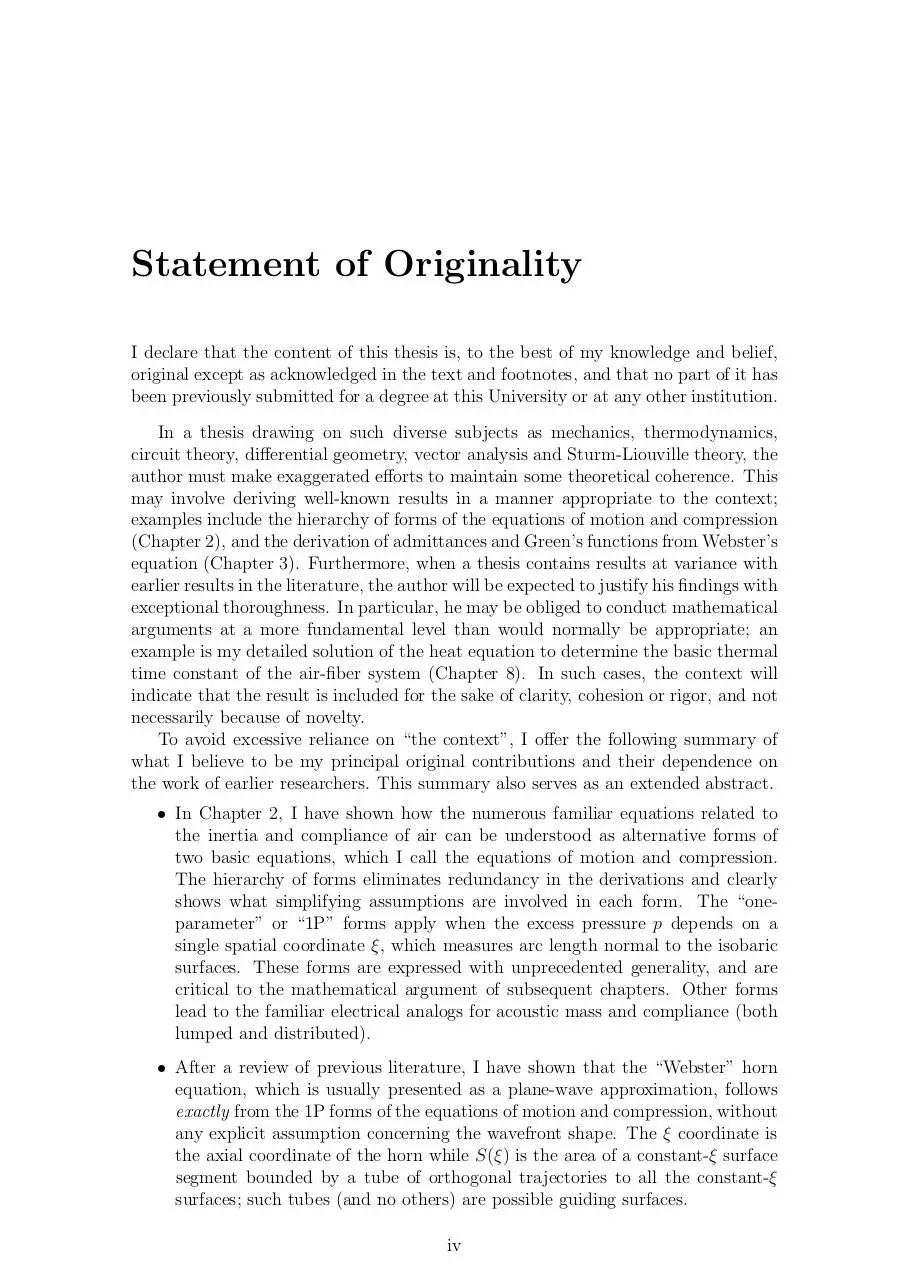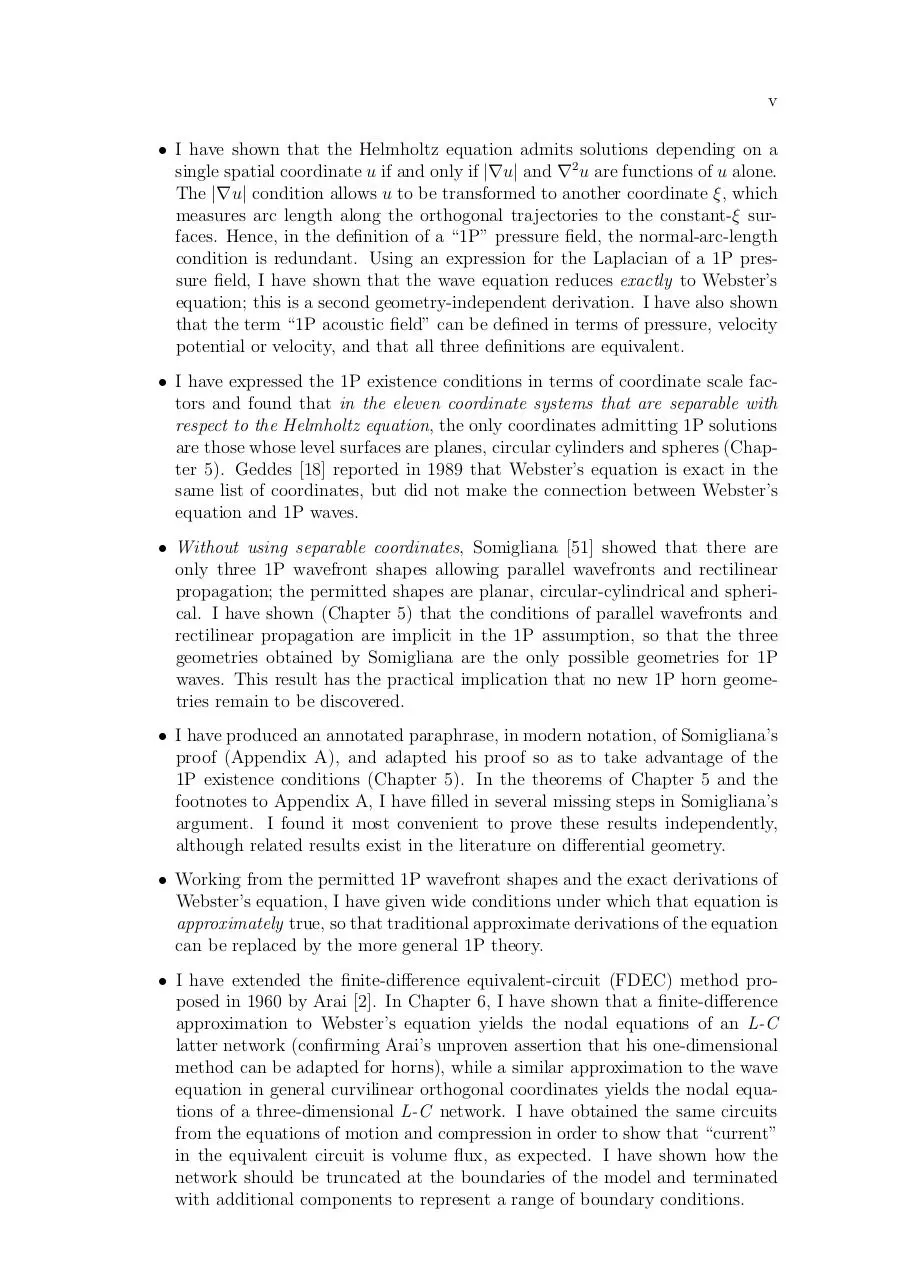# thes .pdf

### File information

This PDF 1.3 document has been generated by TeX output 1997.10.20:1629 / dvipdfm 0.12.6e, Copyright Â© 1998, by Mark A. Wicks, and has been sent on pdf-archive.com on 29/05/2016 at 12:21, from IP address 124.149.x.x. The current document download page has been viewed 1301 times.
File size: 1.3 MB (315 pages).
Privacy: public file

### Document preview

Modeling of
Horns and Enclosures
for Loudspeakers
by
Gavin Richard Putland, BE (Qld)
Department of Electrical and Computer Engineering
University of Queensland

Submitted for the degree of Doctor of Philosophy
December 23, 1994
Revised November 1995
Accepted February 6, 1996.

ii

To my parents,

Frank and Del Putland

Acknowledgments
My interest in horn theory was inspired by Dr E. R. Geddes, whose paper “Acoustic
Waveguide Theory”  raised the questions addressed in Chapters 3 to 5 of this
thesis, suggested some of the answers, and provided many of the related references.
These debts are not diminished by my disagreement with Geddes’ analysis of the
oblate spheroidal waveguide, which he subsequently revised . My paper on oneparameter waves and Webster’s equation  was improved as a result of a personal
communication from Dr Geddes (October 10, 1992), which alerted me to the existence of Fresnel diffraction fringes within the coverage angles of finite horns at high
frequencies.
I wish to thank the Executive Editor and Review Board of the Journal of the
Audio Engineering Society for their patient handling of the complex exchange of
correspondence that followed my “Comments on ‘Acoustic Waveguide Theory’ ” .
I am indebted to an anonymous reviewer of my article “Acoustical Properties of
Air versus Temperature and Pressure”  for correcting my explanation of the effect
of humidity on absorption, and for drawing my attention to ANSI S1.26-1978 ,
which is cited frequently in the final version of the article and in Chapter 9.
My supervisor, Dr Larry Skattebol, provided critical feedback on my three publications and on numerous partial drafts of this thesis. I thank him for his flexibility in
accepting my research proposal, and for his subsequent prudence in curbing my tendency to pursue new lines of inquiry; without his guidance, this already protracted
project would have taken considerably longer. I also thank Prof. Tom Downs and
Dr Nick Shuley, colleagues of Dr Skattebol in the Dept. of Electrical and Computer
Engineering, University of Queensland, for their comments on reference .
The Italian text of Somigliana’s letter  (paraphrased in Appendix A) was
translated by Br. Alan Moss, who was then a graduate student in the Department
of Studies in Religion, University of Queensland.
The Japanese text of Sections 1 to 3 of Arai’s paper  was translated by Adrian
Treloar of the Department of Japanese and Chinese Studies, University of Queensland.
The titles and authors of the major public-domain software used in this project
are as follows. Equivalent circuit simulations were performed using SPICE3 by Tom
Quarles, with the nutmeg user interface by Wayne Christopher. Programs were
compiled by the GNU project C compiler. Most diagrams were drawn using xfig
by Supoj Sutanthavibul et al., converted to LATEX picture commands using fig2dev
by Micah Beck et al., and finally edited as text files. All source files were edited
with jove by Jonathan Payne, and spell-checked with ispell by Pace Willisson et
al. This document was typeset and printed within the Department of Electrical and
Computer Engineering, University of Queensland, using LATEX 2ε by Leslie Lamport.
This project was supported by an Australian Postgraduate Research Award.
iii

Statement of Originality
I declare that the content of this thesis is, to the best of my knowledge and belief,
original except as acknowledged in the text and footnotes, and that no part of it has
been previously submitted for a degree at this University or at any other institution.
In a thesis drawing on such diverse subjects as mechanics, thermodynamics,
circuit theory, differential geometry, vector analysis and Sturm-Liouville theory, the
author must make exaggerated efforts to maintain some theoretical coherence. This
may involve deriving well-known results in a manner appropriate to the context;
examples include the hierarchy of forms of the equations of motion and compression
(Chapter 2), and the derivation of admittances and Green’s functions from Webster’s
equation (Chapter 3). Furthermore, when a thesis contains results at variance with
earlier results in the literature, the author will be expected to justify his findings with
exceptional thoroughness. In particular, he may be obliged to conduct mathematical
arguments at a more fundamental level than would normally be appropriate; an
example is my detailed solution of the heat equation to determine the basic thermal
time constant of the air-fiber system (Chapter 8). In such cases, the context will
indicate that the result is included for the sake of clarity, cohesion or rigor, and not
necessarily because of novelty.
To avoid excessive reliance on “the context”, I offer the following summary of
what I believe to be my principal original contributions and their dependence on
the work of earlier researchers. This summary also serves as an extended abstract.
• In Chapter 2, I have shown how the numerous familiar equations related to
the inertia and compliance of air can be understood as alternative forms of
two basic equations, which I call the equations of motion and compression.
The hierarchy of forms eliminates redundancy in the derivations and clearly
shows what simplifying assumptions are involved in each form. The “oneparameter” or “1P” forms apply when the excess pressure p depends on a
single spatial coordinate ξ, which measures arc length normal to the isobaric
surfaces. These forms are expressed with unprecedented generality, and are
critical to the mathematical argument of subsequent chapters. Other forms
lead to the familiar electrical analogs for acoustic mass and compliance (both
lumped and distributed).
• After a review of previous literature, I have shown that the “Webster” horn
equation, which is usually presented as a plane-wave approximation, follows
exactly from the 1P forms of the equations of motion and compression, without
any explicit assumption concerning the wavefront shape. The ξ coordinate is
the axial coordinate of the horn while S(ξ) is the area of a constant-ξ surface
segment bounded by a tube of orthogonal trajectories to all the constant-ξ
surfaces; such tubes (and no others) are possible guiding surfaces.
iv

v
• I have shown that the Helmholtz equation admits solutions depending on a
single spatial coordinate u if and only if |∇u| and ∇2 u are functions of u alone.
The |∇u| condition allows u to be transformed to another coordinate ξ, which
measures arc length along the orthogonal trajectories to the constant-ξ surfaces. Hence, in the definition of a “1P” pressure field, the normal-arc-length
condition is redundant. Using an expression for the Laplacian of a 1P pressure field, I have shown that the wave equation reduces exactly to Webster’s
equation; this is a second geometry-independent derivation. I have also shown
that the term “1P acoustic field” can be defined in terms of pressure, velocity
potential or velocity, and that all three definitions are equivalent.
• I have expressed the 1P existence conditions in terms of coordinate scale factors and found that in the eleven coordinate systems that are separable with
respect to the Helmholtz equation, the only coordinates admitting 1P solutions
are those whose level surfaces are planes, circular cylinders and spheres (Chapter 5). Geddes  reported in 1989 that Webster’s equation is exact in the
same list of coordinates, but did not make the connection between Webster’s
equation and 1P waves.
• Without using separable coordinates, Somigliana  showed that there are
only three 1P wavefront shapes allowing parallel wavefronts and rectilinear
propagation; the permitted shapes are planar, circular-cylindrical and spherical. I have shown (Chapter 5) that the conditions of parallel wavefronts and
rectilinear propagation are implicit in the 1P assumption, so that the three
geometries obtained by Somigliana are the only possible geometries for 1P
waves. This result has the practical implication that no new 1P horn geometries remain to be discovered.
• I have produced an annotated paraphrase, in modern notation, of Somigliana’s
proof (Appendix A), and adapted his proof so as to take advantage of the
1P existence conditions (Chapter 5). In the theorems of Chapter 5 and the
footnotes to Appendix A, I have filled in several missing steps in Somigliana’s
argument. I found it most convenient to prove these results independently,
although related results exist in the literature on differential geometry.
• Working from the permitted 1P wavefront shapes and the exact derivations of
Webster’s equation, I have given wide conditions under which that equation is
approximately true, so that traditional approximate derivations of the equation
can be replaced by the more general 1P theory.
• I have extended the finite-difference equivalent-circuit (FDEC) method proposed in 1960 by Arai . In Chapter 6, I have shown that a finite-difference
approximation to Webster’s equation yields the nodal equations of an L-C
latter network (confirming Arai’s unproven assertion that his one-dimensional
method can be adapted for horns), while a similar approximation to the wave
equation in general curvilinear orthogonal coordinates yields the nodal equations of a three-dimensional L-C network. I have obtained the same circuits
from the equations of motion and compression in order to show that “current”
in the equivalent circuit is volume flux, as expected. I have shown how the
network should be truncated at the boundaries of the model and terminated
with additional components to represent a range of boundary conditions.

vi

STATEMENT OF ORIGINALITY (WITH EXTENDED ABSTRACT)
• Arai suggested that fibrous damping materials could be handled by using complex values of density and bulk modulus for the air. In Chapter 7, I have
derived expressions for complex density and “complex gamma” (related to
complex bulk modulus) and shown how these quantities can be represented by
introducing additional components into the equivalent circuit.
• An equivalent circuit for a fiber-filled bass enclosure was given by Leach 
in 1989. Leach’s derivation does not use the finite-difference method, is less
rigorous than mine (especially in its treatment of compliance), makes different
assumptions in the determination of complex density, does not use any concept
related to complex bulk modulus, does not use the most appropriate definition
of the thermal time constant (as Leach himself acknowledges), and does not
fully explore the relationships between the possible definitions (the value of the
time constant depends on what conditions are held constant during the heat
transfer). In Chapter 7, I have defined five different thermal time constants, of
which one is useful for deriving the complex gamma and another (which I call
“basic”) is easier to calculate from the specifications of the damping material.
I have shown that two of the five time constants can be read off the equivalent
circuit, and hence expressed all the time constants in terms of the “basic” one,
denoted by τfp , which is the time constant at constant fiber temperature and
constant pressure.
• Values of τfp found by Leach  and Chase  are extremely inaccurate, and
neither author gives a convenient method of calculating the time constant for
arbitrary fiber diameters and packing densities. In Chapter 8, I have rectified
these deficiencies by reworking the solution of the heat equation (finding the
error in Leach’s analysis) and fitting an algebraic formula to the results. The
formula is


d2
(m2 − m0.37 ) ln m+1
τfp ≈
2

where d is the fiber diameter, α is the thermal diffusivity of air, f (not in
the formula) is the fraction of the overall volume occupied by the fiber, and
m = f −1/2 .
• Chapter 9 gives some simple algebraic formulae for calculating α and other
relevant properties of air from the temperature and pressure. This chapter is
mostly a compilation of results from the literature; my only original contributions are some simple curve-fitting and a discussion of errors.
• In Chapter 10, using the results of Chapters 6 to 9, I have constructed a twodimensional finite-difference equivalent-circuit model to predict the frequency
response of a moving-coil loudspeaker in a fiber-filled box. The model incorporates an equivalent circuit of a moving-coil driver, which I have modified so as
to allow the diaphragm to span several volume elements in the interior of the
box. Unlike conventional equivalent-circuit models of loudspeakers, this model
allows for spatial variations of pressure inside the box and shows the effects
of internal resonances on the frequency response. By observing the effects of
omitting selected components from the model, I have found that viscosity is
the dominant mechanism of damping and that, in the cases considered, the
predicted response is not greatly altered by assuming thermal equilibrium. The

vii
latter finding diminishes the significance of, but depends on, the calculation
of the thermal time constant.
• In Chapter 11, I have illustrated and validated the FDEC representation of
a free-air radiation condition in curvilinear coordinates, by applying it to the
classical problem of the circular rigid-piston radiator.
This thesis contains three additional proofs or derivations which I have devised
independently, but which were presumably first discovered by mathematicians of
earlier centuries; in particular, Somigliana  seems to have been familiar with the
first two results as early as 1919. The three passages are:
• the proof that if |∇ξ| = 1, the orthogonal trajectories to the level surfaces of
ξ are straight lines (Theorem 5.1),
• the proof that the only surfaces having constant principal curvatures are
planes, circular cylinders and spheres (Theorem 5.4), and
• the derivation of the so-called “modified Newton method” or “third-order Newton method” for estimating a zero of a non-linear function (Section B.1).

Gavin R. Putland
December 21, 1994

Abstract
It is shown that the “Webster” horn equation is an exact consequence of “oneparameter” or “1P” wave propagation. If a solution of the Helmholtz equation
depends on a single spatial coordinate, that coordinate can be transformed to another coordinate, denoted by ξ, which measures arc length along the orthogonal
trajectories to the constant-ξ surfaces. Webster’s equation, with ξ as the axial coordinate, holds inside a tube of such orthogonal trajectories; the cross-sectional area
in the equation is the area of a constant-ξ cross-section. This derivation of the
horn equation makes no explicit assumption concerning the shape of the wavefronts.
It is subsequently shown, however, that the wavefronts must be planar, circularcylindrical or spherical, so that no new geometries for exact 1P acoustic waveguides
remain to be discovered.
It is shown that if the linearized acoustic field equations are written in arbitrary curvilinear orthogonal coordinates and approximated by replacing all spatial
derivatives by finite-difference quotients, the resulting equations can be interpreted
as the nodal equations of a three-dimensional L-C network. This “finite-difference
equivalent-circuit” or “FDEC” model can be truncated at the boundaries of the simulated region and terminated to represent a wide variety of boundary conditions.
The presence of loosely-packed fibrous damping materials can be represented by using complex values for the density and ratio of specific heats of the medium. These
Two examples of FDEC models are given. The first example predicts the frequency response of a moving-coil loudspeaker in a fiberglass-filled box, showing the
effects of internal resonances. Variations of the model show how the properties
of the fiberglass contribute to the damping of resonances and the shaping of the
frequency response. It is found that viscosity, rather than heat conduction, is the
dominant mechanism of damping. The second example addresses the classical problem of radiation from a circular rigid piston, and confirms that a free-air anechoic
radiation condition with oblique incidence can be successfully represented in the
FDEC model.

viii

Related publications
• G. R. Putland: “Comments on ‘Acoustic Waveguide Theory’ ” (letter), J. Audio Engineering Soc., vol. 39, pp. 469–71 (1991 June). Reply by E. R. Geddes:
pp. 471–2.
• G. R. Putland: “Every One-Parameter Acoustic Field Obeys Webster’s Horn
Equation”, J. Audio Engineering Soc., vol. 41, pp. 435–51 (1993 June).
• G. R. Putland: “Acoustical Properties of Air versus Temperature and Pressure”, J. Audio Engineering Soc., vol. 42, pp. 927–33 (1994 November).
The Journal of the Audio Engineering Society is published in New York. In anticipation of submitting further material to the same journal, the author has adopted
U.S. spellings throughout this thesis.

ixthes.pdf (PDF, 1.3 MB)

### Share on social networks

#### HTML Code

Copy the following HTML code to share your document on a Website or Blog